# Forecast Multivariate Time Series

This example shows how to perform multivariate time series forecasting of data measured from predator and prey populations in a prey crowding scenario. The predator-prey population-change dynamics are modeled using linear and nonlinear time series models. Forecasting performance of these models is compared.

### Data Description

The data is a bivariate time series consisting of 1-predator 1-prey populations (in thousands) collected 10 times a year for 20 years. For more information about the data, see Three Ecological Population Systems: MATLAB and C MEX-File Modeling of Time-Series.

```load PredPreyCrowdingData z = iddata(y,[],0.1,'TimeUnit','years','Tstart',0);```

`z` is an `iddata` object containing two output signals, `y1` and `y2`, which refer to the predator and prey populations, respectively. The `OutputData` property of `z` contains the population data as a 201-by-2 matrix, such that `z.OutputData(:,1)` is the predator population and `z.OutputData(:,2)` is the prey population.

Plot the data.

```plot(z) title('Predator-Prey Population Data') ylabel('Population (thousands)')```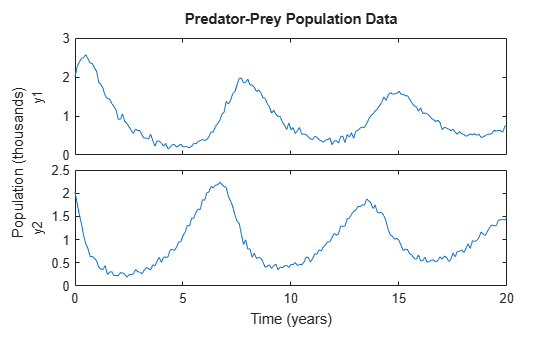The data exhibits a decline in predator population due to crowding.

Use the first half as estimation data for identifying time series models.

`ze = z(1:120);`

Use the remaining data to search for model orders, and to validate the forecasting results.

`zv = z(121:end);`

### Estimate a Linear Model

Model the time series as a linear, autoregressive process. Linear models can be created in polynomial form or state-space form using commands such as `ar` (for scalar time series only), `arx`, `armax`, `n4sid` and `ssest`. Since the linear models do not capture the data offsets (non-zero conditional mean), first detrend the data.

```[zed, Tze] = detrend(ze, 0); [zvd, Tzv] = detrend(zv, 0);```

Identification requires specification of model orders. For polynomial models, you can find suitable orders using the `arxstruc` command. Since `arxstruc `works only on single-output models, perform the model order search separately for each output.

```na_list = (1:10)'; V1 = arxstruc(zed(:,1,:),zvd(:,1,:),na_list); na1 = selstruc(V1,0); V2 = arxstruc(zed(:,2,:),zvd(:,2,:),na_list); na2 = selstruc(V2,0);```

The `arxstruc` command suggests autoregressive models of orders 7 and 8, respectively.

Use these model orders to estimate a multi-variance ARMA model where the cross terms have been chosen arbitrarily.

```na = [na1 na1-1; na2-1 na2]; nc = [na1; na2]; sysARMA = armax(zed,[na nc])```
```sysARMA = Discrete-time ARMA model: Model for output "y1": A(z)y_1(t) = - A_i(z)y_i(t) + C(z)e_1(t) A(z) = 1 - 0.885 z^-1 - 0.1493 z^-2 + 0.8089 z^-3 - 0.2661 z^-4 - 0.9487 z^-5 + 0.8719 z^-6 - 0.2896 z^-7 A_2(z) = 0.3433 z^-1 - 0.2802 z^-2 - 0.04949 z^-3 + 0.1018 z^-4 - 0.02683 z^-5 - 0.2416 z^-6 C(z) = 1 - 0.4534 z^-1 - 0.4127 z^-2 + 0.7874 z^-3 + 0.298 z^-4 - 0.8684 z^-5 + 0.6106 z^-6 + 0.3616 z^-7 Model for output "y2": A(z)y_2(t) = - A_i(z)y_i(t) + C(z)e_2(t) A(z) = 1 - 0.5826 z^-1 - 0.4688 z^-2 - 0.5949 z^-3 - 0.0547 z^-4 + 0.5062 z^-5 + 0.4024 z^-6 - 0.01544 z^-7 - 0.1766 z^-8 A_1(z) = 0.2386 z^-1 + 0.1564 z^-2 - 0.2249 z^-3 - 0.2638 z^-4 - 0.1019 z^-5 - 0.07821 z^-6 + 0.2982 z^-7 C(z) = 1 - 0.1717 z^-1 - 0.09877 z^-2 - 0.5289 z^-3 - 0.24 z^-4 + 0.06555 z^-5 + 0.2217 z^-6 - 0.05765 z^-7 - 0.1824 z^-8 Sample time: 0.1 years Parameterization: Polynomial orders: na=[7 6;7 8] nc=[7;8] Number of free coefficients: 43 Use "polydata", "getpvec", "getcov" for parameters and their uncertainties. Status: Estimated using ARMAX on time domain data "zed". Fit to estimation data: [89.85;90.97]% (prediction focus) FPE: 3.814e-05, MSE: 0.007533 ```

Compute a 10-step-ahead (1 year) predicted output to validate the model over the time span of the estimation data. Since the data was detrended for estimation, you need to specify those offsets for meaningful predictions.

```predOpt = predictOptions('OutputOffset',Tze.OutputOffset'); yhat1 = predict(sysARMA,ze,10, predOpt);```

The `predict` command predicts the response over the time span of measured data and is a tool for validating the quality of an estimated model. The response at time `t` is computed using measured values at times t = 0, ..., t-10.

Plot the predicted response and the measured data.

```plot(ze,yhat1) title('10-step predicted response compared to measured data')```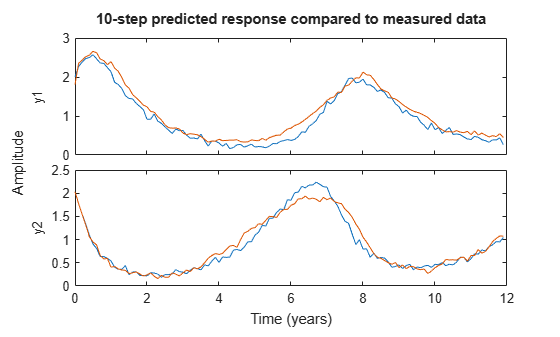Note, the generation of predicted response and plotting it with the measured data, can be automated using the `compare` command.

```compareOpt = compareOptions('OutputOffset',Tze.OutputOffset'); compare(ze,sysARMA,10,compareOpt)```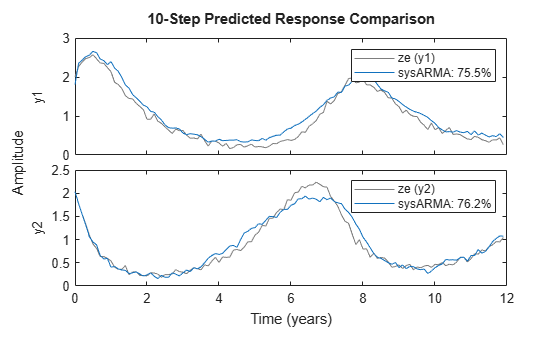The plot generated using `compare` also shows the normalized root mean square (NRMSE) measure of goodness of fit in percent form.

After validating the data, forecast the output of the model `sysARMA` 100 steps (10 years) beyond the estimation data, and calculate output standard deviations.

```forecastOpt = forecastOptions('OutputOffset',Tze.OutputOffset'); [yf1,x01,sysf1,ysd1] = forecast(sysARMA, ze, 100, forecastOpt);```

`yf1` is the forecasted response, returned as an `iddata` object. `yf1.OutputData` contains the forecasted values.

`sysf1` is a system similar to `sysARMA` but is in state-space form. Simulation of `sysf1` using the `sim` command, with initial conditions, `x01`, reproduces the forecasted response, `yf1`.

`ysd1` is the matrix of standard deviations. It measures the uncertainty is forecasting owing to the effect of additive disturbances in the data (as measured by `sysARMA.NoiseVariance`), parameter uncertainty (as reported by `getcov(sysARMA)`) and uncertainties associated with the process of mapping past data to the initial conditions required for forecasting (see `data2state`).

Plot the measured, predicted, and forecasted output for model `sysARMA`.

```t = yf1.SamplingInstants; te = ze.SamplingInstants; t0 = z.SamplingInstants; subplot(1,2,1); plot(t0,z.y(:,1),... te,yhat1.y(:,1),... t,yf1.y(:,1),'m',... t,yf1.y(:,1)+ysd1(:,1),'k--', ... t,yf1.y(:,1)-ysd1(:,1), 'k--') xlabel('Time (year)'); ylabel('Predator population, in thousands'); subplot(1,2,2); plot(t0,z.y(:,2),... te,yhat1.y(:,2),... t,yf1.y(:,2),'m',... t,yf1.y(:,2)+ysd1(:,2),'k--', ... t,yf1.y(:,2)-ysd1(:,2),'k--') % Make the figure larger. fig = gcf; p = fig.Position; fig.Position = [p(1),p(2)-p(4)*0.2,p(3)*1.4,p(4)*1.2]; xlabel('Time (year)'); ylabel('Prey population, in thousands'); legend({'Measured','Predicted','Forecasted','Forecast Uncertainty (1 sd)'},... 'Location','best')```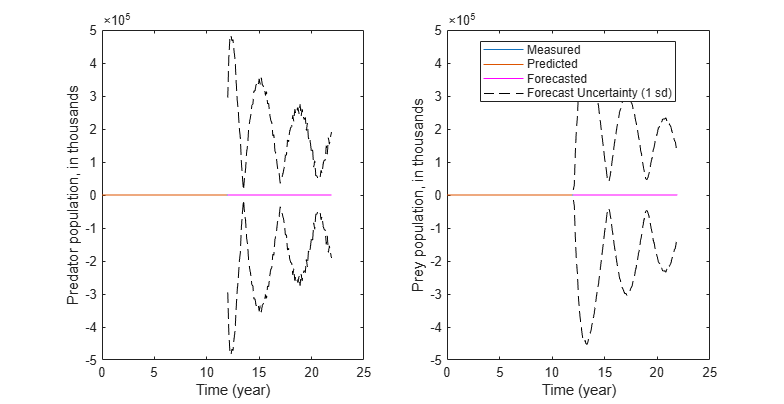The plots show that forecasting using a linear ARMA model (with added handling of offsets) worked somewhat and the results showed high uncertainty compared to the actual populations over the 12-20 years time span. This indicates that the population change dynamics might be nonlinear.

### Estimate Nonlinear Black-Box Models

In this section we extend the black-box identification approach of the previous section by adding nonlinear elements to the model. This is carried out using the Nonlinear ARX modeling framework. Nonlinear ARX models are conceptually similar to the linear ones (such as `sysARMA` above) in that they compute the predicted output using a two-step process:

1. Map the measured signals to a finite-dimensional regressor space.

2. Map the regressors to the predicted output using a static function which can be linear or nonlinear.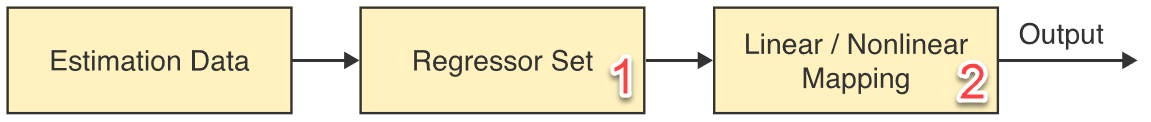Nonlinearities can be added in two places - either in the regressor set (step 1), and/or in the static mapping function (step 2). In this section we explore both of these options. First, we create a linear-in-regressor form of the Nonlinear ARX model where in all the nonlinearities are limited to the regressor definition only; the static mapping function that transforms the regressors into the predicted output is linear (affine, to be exact). Then, we create a model that uses Gaussian Process function as its static mapping function, but keeps its regressors linear.

#### Polynomial AR Model

Create a nonlinear ARX model with 2 outputs and no inputs.

```L = linearRegressor(ze.OutputName,{1,1}); sysNLARX = idnlarx(ze.OutputName, ze.InputName, L, []); sysNLARX.Ts = 0.1; sysNLARX.TimeUnit = 'years';```

`sysNLARX` is a first order nonlinear ARX model that uses no nonlinear function; it predicts the model response using a weighted sum of its first-order regressors.

`getreg(sysNLARX)`
```ans = 2x1 cell {'y1(t-1)'} {'y2(t-1)'} ```

To introduce a nonlinearity function, add polynomial regressors to the model.

Create regressors of order 2 and include cross terms (products of standard regressors listed above). Add those regressors to the model.

```P = polynomialRegressor(ze.OutputName,{1,1},2,false,true); sysNLARX.Regressors(end+1) = P; getreg(sysNLARX)```
```ans = 5x1 cell {'y1(t-1)' } {'y2(t-1)' } {'y1(t-1)^2' } {'y2(t-1)^2' } {'y1(t-1)*y2(t-1)'} ```

Estimate the coefficients (the regressor weightings and the offset) of the model using estimation data, `ze`.

`sysNLARX = nlarx(ze,sysNLARX)`
```sysNLARX = Nonlinear multivariate time series model with 2 outputs Outputs: y1, y2 Regressors: 1. Linear regressors in variables y1, y2 2. Order 2 regressors in variables y1, y2 List of all regressors Output functions: Output 1: None Output 2: None Sample time: 0.1 years Status: Estimated using NLARX on time domain data "ze". Fit to estimation data: [88.34;88.91]% (prediction focus) FPE: 3.265e-05, MSE: 0.01048 ```

Compute a 10-step-ahead predicted output to validate the model.

`yhat2 = predict(sysNLARX,ze,10);`

Forecast the output of the model 100 steps beyond the estimation data.

`yf2 = forecast(sysNLARX,ze,100);`

The standard deviations of the forecasted response are not computed for nonlinear ARX models. This data is unavailable because the parameter covariance information is not computed during estimation of these models.

Plot the measured, predicted, and forecasted outputs.

```t = yf2.SamplingInstants; subplot(1,2,1); plot(t0,z.y(:,1),... te,yhat2.y(:,1),... t,yf2.y(:,1),'m') xlabel('Time (year)'); ylabel('Predator population (thousands)'); title('Prediction Results for ARX Model with Polynomial Regressors') subplot(1,2,2); plot(t0,z.y(:,2),... te,yhat2.y(:,2),... t,yf2.y(:,2),'m') legend('Measured','Predicted','Forecasted') xlabel('Time (year)'); ylabel('Prey population (thousands)');```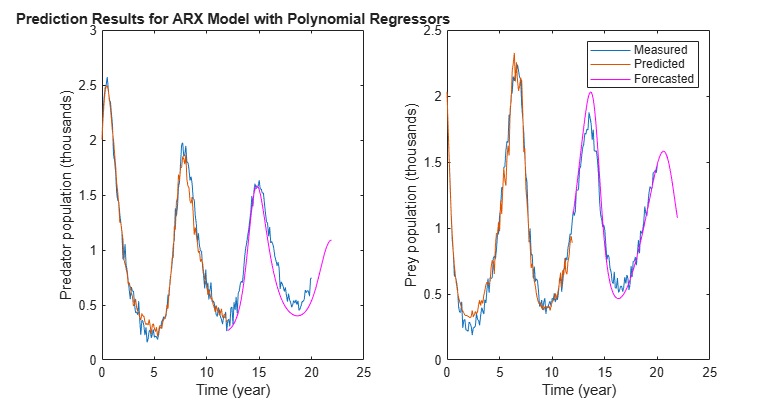The plots show that forecasting using a nonlinear ARX model gave better forecasting results than using a linear model. Nonlinear black-box modeling did not require prior knowledge about the equations governing the data.

Note that to reduce the number of regressors, you can pick an optimal subset of (transformed) variables using principal component analysis (see `pca`) or feature selection (see `sequentialfs`) in the Statistics and Machine Learning Toolbox™.

### Estimate a Gaussian Process Model

The model The model `sysNLARX` uses a linear mapping function; the nonlinearity is contained in the regressors only. A more flexible approach involves picking a non-trivial mapping function, such as a Gaussian Process function (GP). Create a nonlinear ARX model that uses only the linear regressors but adds a Gaussian process function for mapping the regressors to the output.

```% Copy sysNLARX so we don't have to create the model structure again sysGP = sysNLARX; % Remove polynomial regressor set sysGP.Regressors(2) = []; % Replace the linear mapping function by a Gaussian Process (GP) function. % The GP uses a squared exponential kernel by default. GP = idGaussianProcess; sysGP.OutputFcn = GP; disp(sysGP)```
```Nonlinear multivariate time series model with 2 outputs Outputs: y1, y2 Regressors: Linear regressors in variables y1, y2 Output functions: Output 1: Gaussian process function using a SquaredExponential kernel. Output 2: Gaussian process function using a SquaredExponential kernel. Sample time: 0.1 years Status: Estimated using NLARX with prediction focus ```

You now have a template model sysGP that is based on linear regressors and a GP mapping function. Its parameters are the two coefficients of its squared exponential kernel. Use `nlarx` to estimate these parameters.

`sysGP = nlarx(ze, sysGP)`
```sysGP = Nonlinear multivariate time series model with 2 outputs Outputs: y1, y2 Regressors: Linear regressors in variables y1, y2 List of all regressors Output functions: Output 1: Gaussian process function using a SquaredExponential kernel. Output 2: Gaussian process function using a SquaredExponential kernel. Sample time: 0.1 years Status: Estimated using NLARX on time domain data "ze". Fit to estimation data: [88.07;88.84]% (prediction focus) FPE: 3.369e-05, MSE: 0.01083 ```
`getpvec(sysGP)`
```ans = 10×1 -0.6671 0.0196 0.9558 0.9445 0.0261 -0.0221 -0.5752 0.8479 1.1883 0.0447 ```

As before, compute the predicted and forecasted responses and plot results.

Compute a 10-step-ahead predicted output to validate the model.

`yhat3 = predict(sysGP,ze,10);`

Forecast the output of the model 100 steps beyond the estimation data.

`yf3 = forecast(sysGP,ze,100);`

Plot the measured, predicted, and forecasted outputs.

```t = yf3.SamplingInstants; subplot(1,2,1); plot(t0,z.y(:,1),... te,yhat3.y(:,1),... t,yf3.y(:,1),'m') xlabel('Time (year)'); ylabel('Predator population (thousands)'); title('Prediction Results for Gaussian Process Model') subplot(1,2,2); plot(t0,z.y(:,2),... te,yhat3.y(:,2),... t,yf3.y(:,2),'m') legend('Measured','Predicted','Forecasted') xlabel('Time (year)'); ylabel('Prey population (thousands)');```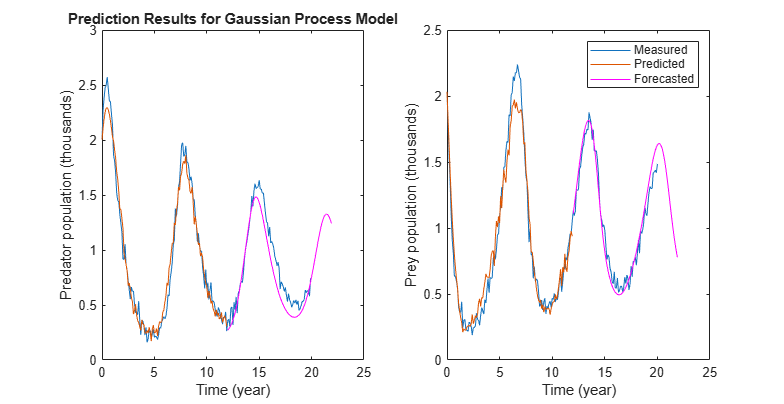The results show improved accuracy over the linear model. An advantage of using GP based models is that they employ very few parameters, as opposed to other forms such as Wavelet Networks (`idWaveletNetwork`) or Sigmoid Networks (`idSigmoidNetwork`). This makes them easy to train with low uncertainty in their parameter estimates.

### Estimate a Nonlinear Grey-Box Model

If you have prior knowledge of the system dynamics, you can fit the estimation data using a nonlinear grey-box model. Knowledge about the nature of the dynamics can, in general, help improve the model quality and thus the forecasting accuracy. For the predator-prey dynamics, the changes in the predator (`y1`) and prey (`y2`) population can be represented as:

`${\underset{}{\overset{˙}{y}}}_{1}\left(t\right)={p}_{1}*{y}_{1}\left(t\right)+{p}_{2}*{y}_{2}\left(t\right)*{y}_{1}\left(t\right)$`

`${\underset{}{\overset{˙}{y}}}_{2}\left(t\right)={p}_{3}*{y}_{2}\left(t\right)-{p}_{4}*{y}_{1}\left(t\right)*{y}_{2}\left(t\right)-{p}_{5}*{y}_{2}\left(t{\right)}^{2}$`

For more information about the equations, see Three Ecological Population Systems: MATLAB and C MEX-File Modeling of Time-Series.

Construct a nonlinear grey-box model based on these equations.

Specify a file describing the model structure for the predator-prey system. The file specifies the state derivatives and model outputs as a function of time, states, inputs, and model parameters. The two outputs (predator and prey populations) are chosen as states to derive a nonlinear state-space description of the dynamics.

`FileName = 'predprey2_m';`

Specify the model orders (number of outputs, inputs, and states)

`Order = [2 0 2];`

Specify the initial values for the parameters ${p}_{1}$, ${p}_{2}$, ${p}_{3}$, ${p}_{4}$, and ${p}_{5}$, and indicate that all parameters are to be estimated. Note that the requirement to specify initial guesses for parameters did not exist when estimating the black box models `sysARMA` and `sysNLARX`.

```Parameters = struct('Name',{'Survival factor, predators' 'Death factor, predators' ... 'Survival factor, preys' 'Death factor, preys' ... 'Crowding factor, preys'}, ... 'Unit',{'1/year' '1/year' '1/year' '1/year' '1/year'}, ... 'Value',{-1.1 0.9 1.1 0.9 0.2}, ... 'Minimum',{-Inf -Inf -Inf -Inf -Inf}, ... 'Maximum',{Inf Inf Inf Inf Inf}, ... 'Fixed',{false false false false false});```

Similarly, specify the initial states of the model, and indicate that both initial states are to be estimated.

```InitialStates = struct('Name',{'Predator population' 'Prey population'}, ... 'Unit',{'Size (thousands)' 'Size (thousands)'}, ... 'Value',{1.8 1.8}, ... 'Minimum', {0 0}, ... 'Maximum',{Inf Inf}, ... 'Fixed',{false false});```

Specify the model as a continuous-time system.

`Ts = 0;`

Create a nonlinear grey-box model with specified structure, parameters, and states.

`sysGrey = idnlgrey(FileName,Order,Parameters,InitialStates,Ts,'TimeUnit','years');`

Estimate the model parameters.

```sysGrey = nlgreyest(ze,sysGrey); present(sysGrey)```
``` sysGrey = Continuous-time nonlinear grey-box model defined by 'predprey2_m' (MATLAB file): dx/dt = F(t, x(t), p1, ..., p5) y(t) = H(t, x(t), p1, ..., p5) + e(t) with 0 input(s), 2 state(s), 2 output(s), and 5 free parameter(s) (out of 5). States: Initial value x(1) Predator population(t) [Size (thou..] xinit@exp1 2.01325 (estimated) in [0, Inf] x(2) Prey population(t) [Size (thou..] xinit@exp1 1.99687 (estimated) in [0, Inf] Outputs: y(1) y1(t) y(2) y2(t) Parameters: Value Standard Deviation p1 Survival factor, predators [1/year] -0.995895 0.0125269 (estimated) in [-Inf, Inf] p2 Death factor, predators [1/year] 1.00441 0.0129368 (estimated) in [-Inf, Inf] p3 Survival factor, preys [1/year] 1.01234 0.0135413 (estimated) in [-Inf, Inf] p4 Death factor, preys [1/year] 1.01909 0.0121026 (estimated) in [-Inf, Inf] p5 Crowding factor, preys [1/year] 0.103244 0.0039285 (estimated) in [-Inf, Inf] Status: Termination condition: Change in cost was less than the specified tolerance.. Number of iterations: 6, Number of function evaluations: 7 Estimated using Solver: ode45; Search: lsqnonlin on time domain data "ze". Fit to estimation data: [91.21;92.07]% FPE: 8.613e-06, MSE: 0.005713 More information in model's "Report" property. ```

Compute a 10-step-ahead predicted output to validate the model.

`yhat4 = predict(sysGrey,ze,10);`

Forecast the output of the model 100 steps beyond the estimation data, and calculate output standard deviations.

`[yf4,x04,sysf4,ysd4] = forecast(sysGrey,ze,100);`

Plot the measured, predicted, and forecasted outputs.

```t = yf4.SamplingInstants; subplot(1,2,1); plot(t0,z.y(:,1),... te,yhat4.y(:,1),... t,yf4.y(:,1),'m',... t,yf4.y(:,1)+ysd4(:,1),'k--', ... t,yf4.y(:,1)-ysd4(:,1),'k--') xlabel('Time (year)'); ylabel('Predator population (thousands)'); title('Prediction Results for Grey-Box Model') subplot(1,2,2); plot(t0,z.y(:,2),... te,yhat4.y(:,2),... t,yf4.y(:,2),'m',... t,yf4.y(:,2)+ysd4(:,2),'k--', ... t,yf4.y(:,2)-ysd4(:,2),'k--') legend('Measured','Predicted','Forecasted','Forecast uncertainty (1 sd)') xlabel('Time (years)'); ylabel('Prey population (thousands)');```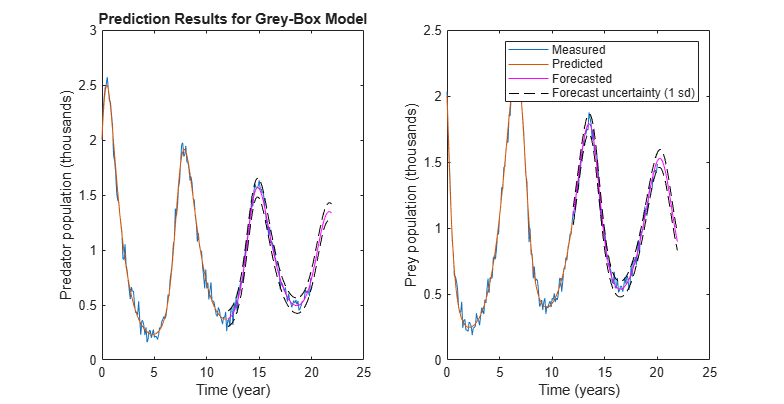The plots show that forecasting using a nonlinear grey-box model gave good forecasting results and low forecasting output uncertainty.

### Compare Forecasting Performance

Compare the forecasted response obtained from the identified models, `sysARMA`, `sysNLARX`, and `sysGrey`. The first two are discrete-time models and `sysGrey` is a continuous-time model.

```clf plot(z,yf1,yf2,yf3,yf4) legend({'Measured','ARMA','Polynomial AR','GP','Grey-Box'}) title('Forecasted Responses') xlim([7 23])```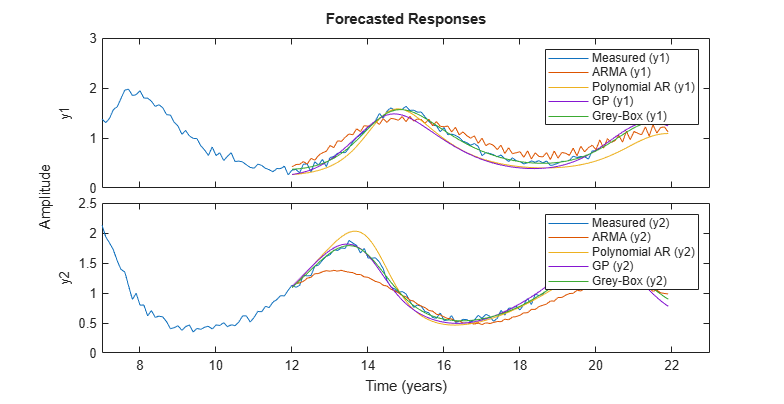Forecasting with a nonlinear ARX model gave better results than forecasting with a linear model. Inclusion of the knowledge of the dynamics in the nonlinear grey-box model further improved the reliability of the model and therefore the forecasting accuracy.

Note that the equations used in grey-box modeling are closely related to the polynomial regressors used by the Nonlinear ARX model. If you approximate the derivatives in the governing equations by first-order differences, you will get equations similar to:

`${y}_{1}\left(t\right)={s}_{1}*{y}_{1}\left(t-1\right)+{s}_{2}*{y}_{1}\left(t-1\right)*{y}_{2}\left(t-1\right)$`

`${y}_{2}\left(t\right)={s}_{3}*{y}_{2}\left(t-1\right)-{s}_{4}*{y}_{1}\left(t-1\right)*{y}_{2}\left(t-1\right)-{s}_{5}*{y}_{2}\left(t-1{\right)}^{2}$`

Where, ${s}_{1},...,{s}_{5}$ are some parameters related to the original parameters ${p}_{1},...,{p}_{5}$ and to the sample time used for differencing. These equations suggest that only 2 regressors are needed for the first output (y1(t-1) and *y1(t-1)*y2(t-1)) and 3 for the second output when constructing the Nonlinear ARX model. Even in absence of such prior knowledge, linear-in-regressor model structures employing polynomial regressors remain a popular choice in practice.

Forecast the values using the nonlinear grey-box model over 200 years.

`[yf5,~,~,ysd5] = forecast(sysGrey, ze, 2000);`

Plot the latter part of the data (showing 1 sd uncertainty)

```t = yf5.SamplingInstants; N = 700:2000; subplot(1,2,1); plot(t(N), yf5.y(N,1), 'm',... t(N), yf5.y(N,1)+ysd5(N,1), 'k--', ... t(N), yf5.y(N,1)-ysd5(N,1), 'k--') xlabel('Time (year)'); ylabel('Predator population (thousands)'); title('Forecasted Population after 200 Years') ax = gca; ax.YLim = [0.8 1]; ax.XLim = [82 212]; subplot(1,2,2); plot(t(N),yf5.y(N,2),'m',... t(N),yf5.y(N,2)+ysd5(N,2),'k--', ... t(N),yf5.y(N,2)-ysd5(N,2),'k--') legend('Forecasted population','Forecast uncertainty (1 sd)') xlabel('Time (years)'); ylabel('Prey population (thousands)'); ax = gca; ax.YLim = [0.9 1.1]; ax.XLim = [82 212];```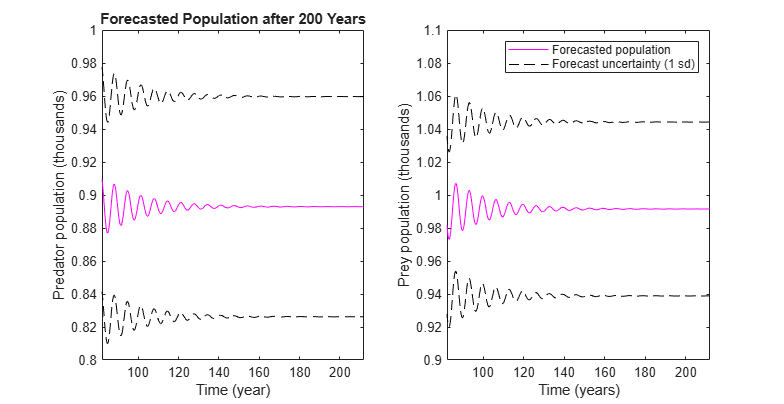The plot show that the predatory population is forecasted to reach a steady-state of approximately 890 and the prey population is forecasted to reach 990.

## SupportGet trial now